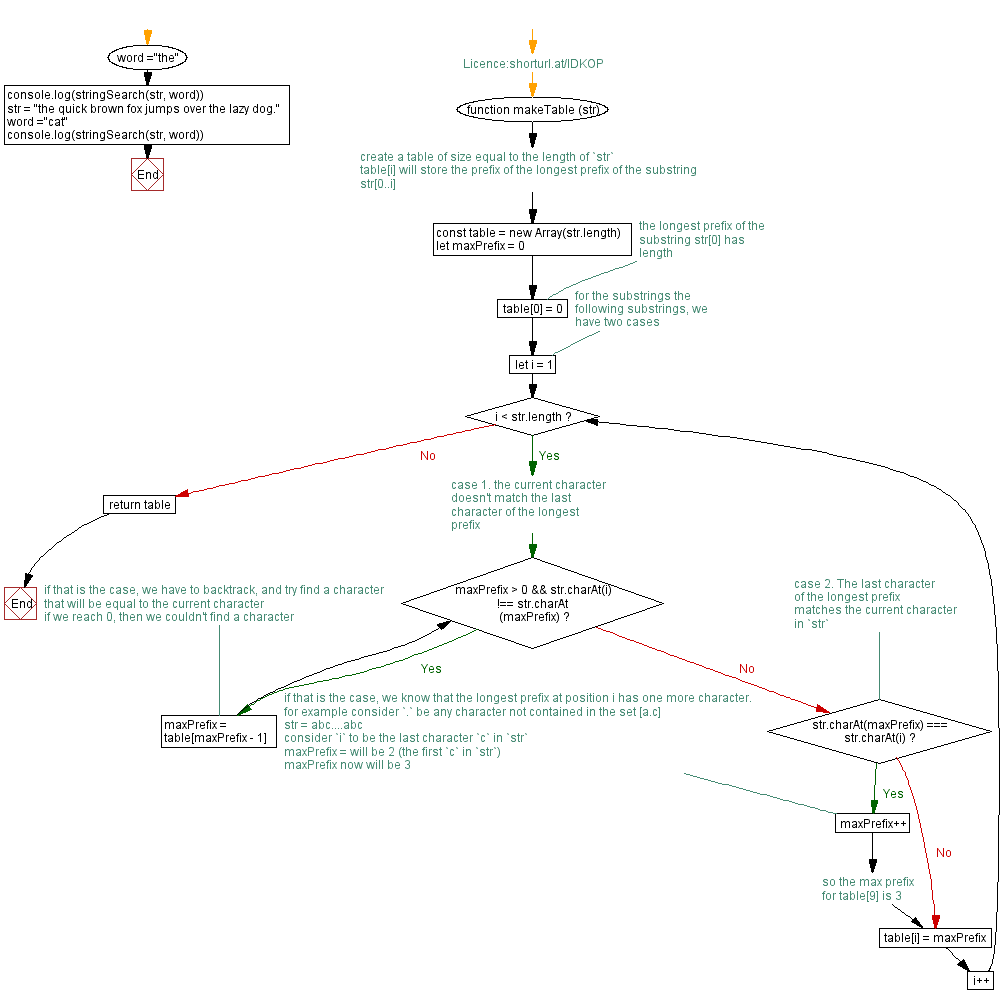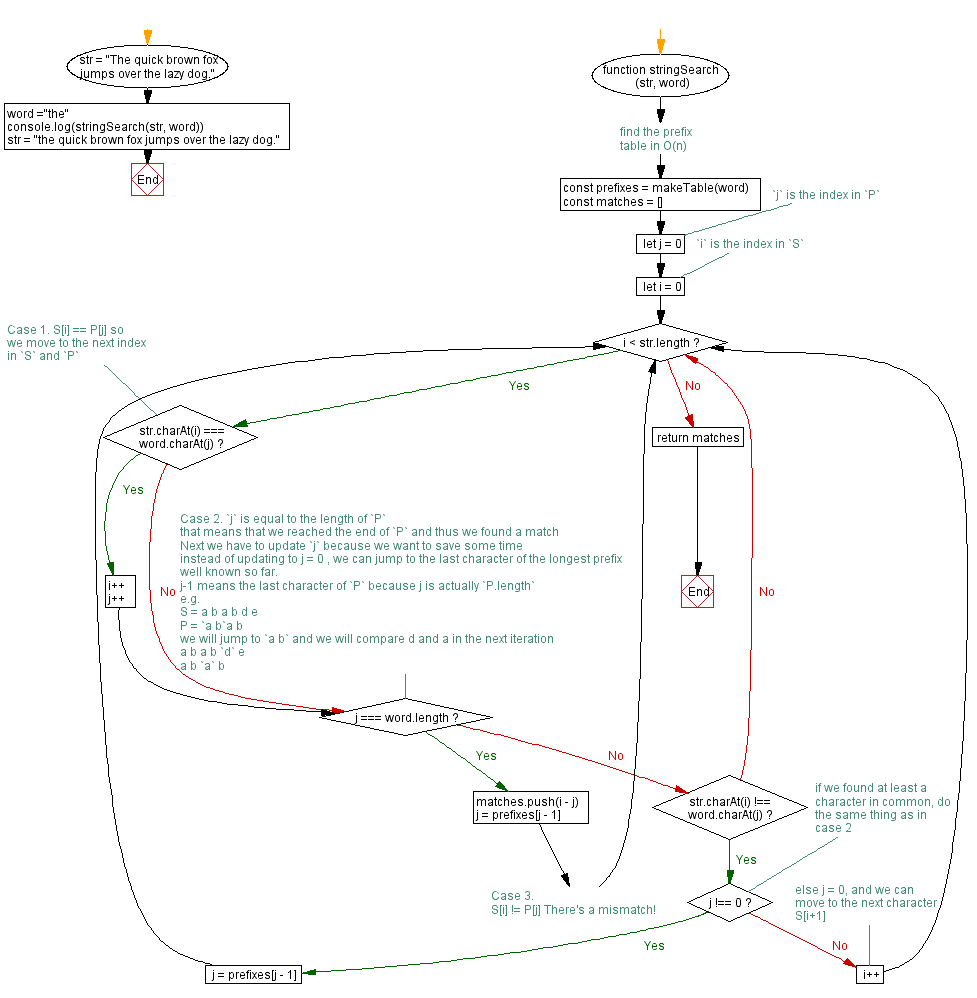# JavaScript - Index position of a word within a string

## JavaScript Searching Algorithm: Exercise-1 with Solution

Write a JavaScript program to find all the index positions of a given word within a given string.

Test Data:
( "The quick brown fox jumps over the lazy dog.", "the") -> 
( "the quick brown fox jumps over the lazy dog.", "the") -> [0, 31]
( "the quick brown fox jumps over the lazy dog.", "cat") -> []

Sample Solution:

JavaScript Code:

``````//Licence:shorturl.at/lDKOP
function makeTable (str) {
// create a table of size equal to the length of `str`
// table[i] will store the prefix of the longest prefix of the substring str[0..i]
const table = new Array(str.length)
let maxPrefix = 0
// the longest prefix of the substring str has length
table = 0

// for the substrings the following substrings, we have two cases
for (let i = 1; i < str.length; i++) {
// case 1. the current character doesn't match the last character of the longest prefix
while (maxPrefix > 0 && str.charAt(i) !== str.charAt(maxPrefix)) {
// if that is the case, we have to backtrack, and try find a character  that will be equal to the current character
// if we reach 0, then we couldn't find a character
maxPrefix = table[maxPrefix - 1]
}
// case 2. The last character of the longest prefix matches the current character in `str`
if (str.charAt(maxPrefix) === str.charAt(i)) {
// if that is the case, we know that the longest prefix at position i has one more character.
// for example consider `.` be any character not contained in the set [a.c]
// str = abc....abc
// consider `i` to be the last character `c` in `str`
// maxPrefix = will be 2 (the first `c` in `str`)
// maxPrefix now will be 3
maxPrefix++
// so the max prefix for table is 3
}
table[i] = maxPrefix
}
return table
}
function stringSearch (str, word) {
// find the prefix table in O(n)
const prefixes = makeTable(word)
const matches = []
// `j` is the index in `P`
let j = 0
// `i` is the index in `S`
let i = 0
while (i < str.length) {
// Case 1.  S[i] == P[j] so we move to the next index in `S` and `P`
if (str.charAt(i) === word.charAt(j)) {
i++
j++
}
// Case 2.  'j' is equal to the length of 'P'
// that means that we reached the end of 'P' and thus we found a match
// Next we have to update 'j' because we want to save some time
// instead of updating to j = 0 , we can jump to the last character of the longest prefix well known so far.
// j-1 means the last character of 'P' because j is actually 'P.length'
// e.g.
// S =  a b a b d e
// P = 'a b'a b
// we will jump to `a b` and we will compare d and a in the next iteration
// a b a b 'd' e
//     a b 'a' b
if (j === word.length) {
matches.push(i - j)
j = prefixes[j - 1]
// Case 3.
// S[i] != P[j] There's a mismatch!
} else if (str.charAt(i) !== word.charAt(j)) {
// if we  found at least a character in common, do the same thing as in case 2
if (j !== 0) {
j = prefixes[j - 1]
} else {
// else j = 0, and we can move to the next character S[i+1]
i++
}
}
}
return matches
}
str = "The quick brown fox jumps over the lazy dog."
word ="the"
console.log(stringSearch(str, word))
str = "the quick brown fox jumps over the lazy dog."
word ="the"
console.log(stringSearch(str, word))
str = "the quick brown fox jumps over the lazy dog."
word ="cat"
console.log(stringSearch(str, word))
```
```

Sample Output:

```
[0,31]
[]
```

Flowchart:Live Demo:

See the Pen javascript-searching-algorithm-exercise-1 by w3resource (@w3resource) on CodePen.

* To run the code mouse over on Result panel and click on 'RERUN' button.*

Improve this sample solution and post your code through Disqus

What is the difficulty level of this exercise?

Test your Programming skills with w3resource's quiz.

﻿